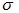# Types of Bending Stress

1. Pure Bending Stress

Bending will be called as pure bending when it occurs solely because of coupling on its end. In that case there is no chance of shear stress in the beam. But, the stress that will propagate in the beam as a result will be known as normal stress. Normal stress because it not causing any damages to beam. As shown below in the picture.

FIg 2: Pure Bending stresses are those that results beacuse of beam self load only.

2. Simple Bending Stress

Bending will be called as simple bending when it occurs because of beam self-load and external load. This type of bending is also known as ordinary bending and in this type of bending results both shear stress and normal stress in the beam. As shown below in the figure.

Fig 3: Simple Bending Stress

Formula for Flexural Stress

Where,

M= bending moment

I = moment of inertia of the section about the bending axis.=fibre stress at a distance ‘y’ from the centroidal/neutral axis.

E = Young’s Modulus of the material of the beam.

R = radius of curvature of the bent beam.

If y is replaced by c, the distance to remotest element, then

Where, Z= section modulus and is given by, Z = I/c